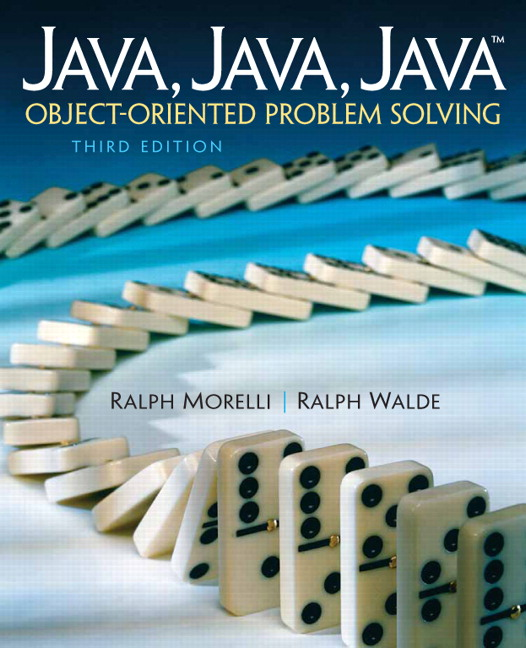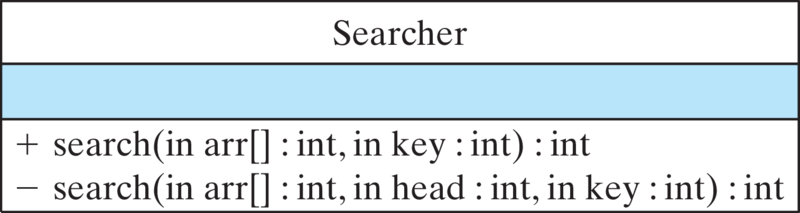# Java, Java, Java: Object-Oriented Problem Solving, 2022E

## Section12.4Recursive Array Processing

Like strings, arrays also have a recursive structure. Just as each substring of a string is similar to the string as a whole, each portion of an array is similar to the array as a whole. Similarly, just as a string can be divided into a head and a tail, an array can be divided into its head, the first element, and its tail, the rest of its elements (Figure 12.4.1). Because the tail of an array is itself an array, it satisfies the self-similarity principle. Therefore, arrays have all the appropriate characteristics that make them excellent candidates for recursive processing.

### Subsection12.4.1Recursive Sequential Search

Let’s start by developing a recursive version of the sequential search algorithm that we discussed in Chapter 9. Recall that the sequential search method takes two parameters: the array being searched and the key, or target value, being searched for. If the key is found in the array, the method returns its index. If the key is not found, the method returns $$-1\text{,}$$ thereby indicating that the key was not contained in the array. The iterative version of this method has the following general form:
/**
* Performs a sequential search of an integer array
* @param arr is the array of integers
* @param key is the element being searched for
* @return the key's index is returned if the key is
*  found otherwise -1 is returned
* Pre:  arr is not null
* Post: either -1 or the key's index is returned
*/
public int sequentialSearch(int arr[], int key) {
return -1;           // failure if this is reached
}

If we divide the array into its head and tail, then one way to describe a recursive search algorithm is as follows:
This algorithm clearly resembles the approach we used in recursive string processing: Perform some operation on the head of the array and recurse on the tail of the array.
The challenge in developing this algorithm is not so much knowing what to do but knowing how to represent concepts like the head and the tail of the array. For strings, we had methods such as s.charAt(0) to represent the head of the string and s.substring(1) to represent the string’s tail. For an array named arr, the expression arr represents the head of the array. Unfortunately, we have no method comparable to the substring() method for strings that lets us represent the tail of the array.
To help us out of this dilemma, we can use an integer parameter to represent the head of the array. Let’s have the int parameter, head, represent the current head of the array (Figure 12.4.3). Then $$head+1$$ represents the start of the tail, and arr.length-1 represents the end of the tail. Our method will always be passed a reference to the whole array, but it will restrict the search to the portion of the array starting at head. If we let head vary from 0 to arr.length on each recursive call, the method will recurse through the array in head/tail fashion, searching for the key. The method will stop when head = arr.length.
This leads to the definition for recursive search shown in Listing 12.4.5. Note that the recursive search method takes three parameters: the array to be searched, arr, the key being sought, and an integer head that gives the starting location for the search. The algorithm is bounded when head = arr.length. In effect, this is like saying the recursion should stop when we have reached a tail that contains 0 elements. This underscores the point we made earlier about the importance of parameters in designing recursive methods. Here the head parameter serves as the recursion parameter. It controls the progress of the recursion.
Note also that for the search algorithm we need two base cases. One represents the successful case, where the key is found in the array. The other represents the unsuccessful case, which comes about after we have looked at every possible head in the array and not found the key. This case will arise through exhaustion—that is, when we have exhausted all possible locations for the key.

### Subsection12.4.2Information Hiding

Note that in order to use the search() method, you would have to know that you must supply a value of 0 as the argument for the head parameter. This is not only awkward but also impractical. After all, if we want to search an array, we just want to pass two arguments, the array and the key we’re searching for. It’s unreasonable to expect users of a method to know that they also have to pass 0 as the head in order to get the recursion started. This design is also prone to error, because it’s quite easy for a mistake to be made when the method is called.
For this reason, it is customary to provide a nonrecursive interface to the recursive method. The interface hides the fact that a recursive algorithm is being used, but this is exactly the kind of implementation detail that should be hidden from the user. This is an example of the principle of information hiding that we introduced in Chapter 0. A more appropriate design would make the recursive method a private method that’s called by the public method, as shown Figure 12.4.7 and implemented in the Searcher class (Listing 12.4.8).Figure 12.4.7. The public search() method serves as an interface to the private recursive method, search(). Note that the methods have different signatures.

### Subsection12.4.3Recursive Selection Sort

Next we want you to think back to Chapter 9, where we introduced the selection sort algorithm. To review the concept, suppose you have a deck of 52 cards. Lay them out on a table, face up, one card next to the other. Starting at the last card, look through the deck, from last to first, find the largest card and exchange it with the last card. Then go through the deck again starting at the next to the last card, find the next largest card, and exchange it with the next to the last card. Go to the next card, and so on. If you repeat this process 51 times, the deck will be completely sorted.
Let’s design a recursive version of this algorithm. The algorithm we just described is like a head-and-tail algorithm in reverse, where the last card in the deck is like the head, and the cards before it are like the tail. After each pass or recursion, the last card will be in its proper location, and the cards before it will represent the unsorted portion of the deck. If we use parameter to represent last, then it will be moved one card to the left at each level of the recursion.
Figure 12.4.11 illustrates this process for an array of integers. The base case is reached when the last parameter is pointing to the first element in the array. An array with one element in it is already sorted. It needs no rearranging. The recursive case involves searching an ever-smaller portion of the array. This is represented in our design by moving last down one element to the left.
ListingA 12.4.12 provides a partial implementation of selection sort for an array of int. In this definition, the array is one parameter. The second parameter, int last, defines that portion of the array, from right to left, that is yet to be sorted. On the first call to this method, last will be arr.length - 1. On the second, it will be arr.length - 2, and so on. When last gets to be 0, the array will be sorted. Thus, in terms of the card deck analogy, last represents the last card in the unsorted portion of the deck.
Note how simply the selectionSort() method can be coded. Of course, this is because we have used separate methods to handle the tasks of finding the largest element and swapping the last element and the largest. This not only makes sense in terms of the divide-and-conquer principle, but we also already defined a swap() method in Chapter 9. So here is a good example of reusing code:
/**
* swap(arr, el1 el2) swaps el1 and el2 in the array, arr
* Pre: arr is non null, 0 <= el1 < arr.length,
0 <= el2 < arr.length
* Post: the locations of el1 and el2 are swapped in arr
*/
private void swap(int arr[], int el1, int el2)  {
int temp = arr[el1]; // Assign the first element to temp
arr[el1] = arr[el2]; // Overwrite first with second
arr[el2] = temp;     // Overwrite second with first(temp)
} // swap()

The definition of the findMax() method is left as a self-study exercise.

#### ExercisesSelf-Study Exercises

##### 1.Find Max.
Define an iterative version of the findMax(arr,N) method that is used in selectionSort(). Its goal is to return the location (index) of the largest integer between arr and arr[N].
##### 2.Selection sort.
As in the case of the search() method, we need to provide a public interface to the recursive selectionSort() method. We want to enable the user to sort an array just by calling sort(arr), where arr is the name of the array to be sorted. Define the sort() method.